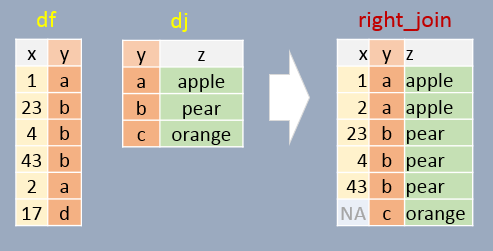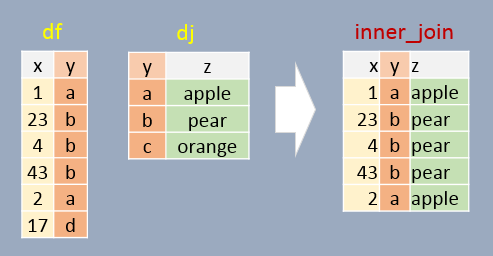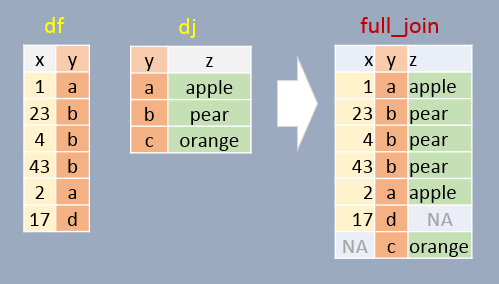dplyr
1.0.4

We can use dplyr’s join operations to join elements from one table to another table. Four such functions (with differing behaviors) are left_join, right_join, inner_join, and full join.

To demonstrate these functions, we’ll be joining two dataframes: df and dj.

library(dplyr)

df <- data.frame( x = c(1, 23, 4, 43, 2, 17),
y = c("a", "b", "b", "b", "a", "d"),
stringsAsFactors = FALSE)
df
   x y
1  1 a
2 23 b
3  4 b
4 43 b
5  2 a
6 17 d
dj <- data.frame( z = c("apple", "pear", "orange"),
y = c("a", "b", "c"),
stringsAsFactors = FALSE)
dj
       z y
1  apple a
2   pear b
3 orange c

In the examples that follow, we will join both tables by the common column y.

# Left join

In this example, if a join element in df does not exist in dj, NA will be assigned to column z. In other words, all elements in df will exist in the output regardless if a matching element is found in dj. Note that the output is sorted in the same order as df (the left table).

left_join(df, dj, by="y")
   x y     z
1  1 a apple
2 23 b  pear
3  4 b  pear
4 43 b  pear
5  2 a apple
6 17 d  <NA># Right join

If a join element in df does not exist in dj, that element is removed from the output. A few additional important notes follow:

• All elements in dj appear at least once in the output (even if they don’t have a match in df in which case an NA value is added),
• The output table is sorted in the order in which the y elements appear in dj.
• Element y will appear as many times as there matching ys in df.
right_join(df, dj, by="y")
   x y      z
1  1 a  apple
2 23 b   pear
3  4 b   pear
4 43 b   pear
5  2 a  apple
6 NA c orange# Inner join

In this example, only matching elements in both df and dj are saved in the output.

inner_join(df, dj, by="y")
   x y     z
1  1 a apple
2 23 b  pear
3  4 b  pear
4 43 b  pear
5  2 a apple# Full join

In this example, all elements in both df and dj are present in the output. For non-matching pairs, NA values are supplied.

full_join(df, dj, by="y")
   x y      z
1  1 a  apple
2 23 b   pear
3  4 b   pear
4 43 b   pear
5  2 a  apple
6 17 d   <NA>
7 NA c orange# Joins in a piping operation

The afrementioned joining functions can be used with pipes. For example:

df %>%
left_join(dj, by = "y")
   x y     z
1  1 a apple
2 23 b  pear
3  4 b  pear
4 43 b  pear
5  2 a apple
6 17 d  <NA>

# A note about column names

If the common columns in both tables have different names, you will need to modify the by = argument as by = c("left_col" = "right_col"). For example,

library(dplyr)

df <- data.frame( x = c(1, 23, 4, 43, 2, 17),
y1 = c("a", "b", "b", "b", "a", "d"),
stringsAsFactors = FALSE)

dj <- data.frame( z = c("apple", "pear", "orange"),
y2 = c("a", "b", "c"),
stringsAsFactors = FALSE)

left_join(df, dj, by = c("y1" = "y2"))
   x y1     z
1  1  a apple
2 23  b  pear
3  4  b  pear
4 43  b  pear
5  2  a apple
6 17  d  <NA>`